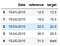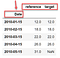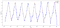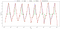# Imputing the Time-Series Using Python

Time series are an important form of indexed data found in stocks data, climate datasets, and many other time-dependent data forms. Due to its time-dependency, time series are subject to have missing points due to problems in reading or recording the data.

To apply machine learning models effectively, the time series has to be continuous, as most of the ML models are not designed to deal with missing values. Hence, the rows with missing data should be either dropped or filled with appropriate values.

In time-independent data (non-time-series), a common practice is to fill the gaps with the mean or median value of the field. However, this is not applicable in the time series. To understand the reason, let’s consider a temperature dataset. The temperature value of February is very far from its value in July. This is also applicable to sales dataset that has some seasons with high sales and others with low or regular sales. So the imputation method should be dependent on time.

To prove this assumption, let’s take an example and solve it in python.

Loading and preparing the dataset

Import the required libraries, and read the dataThe head of the dataset, notice the date is a normal column.

The dataset contains three columns, `Date,`the date in `dd-mm-yyyy` format; `reference` the temperature column with no missing data for reference; and `target` the temperature column with random missing points.

The first step is to set the index of the dataframe to be the `Date` columnThe `Date` column is now an index

For charting purposes, we will add a column that contains the missing values only.

Notice that we have 21 missing points out of 96 total points.

Have a look at the data `df.plot(style=['k--', 'bo-', 'r*'], figsize=(20, 10));`The temperature data with the available points in blue and missing points in red

Trying to impute using the mean/median values.

I will create a column for each tested method to compare the values later.

Trying to impute using the rolling average.

Imputing using interpolation with different methods

Scoring the results and see which is better

The result shows that the `'time'` method as well as the `'slinear'` method produces the closest values to the original values, while the rolling mean and median produces very low values of r^2.

To plot the data after imputation.The `time` interpolation is the best method for time series.

Some limitations

1. For the `time` interpolation to succeed, the dataframe must have the index in Date format with intervals of 1 day or more (daily, monthly, …); however, it will not work for time-based data, like hourly data.
2. if it is important to use a different index for the dataframe, use `reset_index().set_index('Date')`, do the interpolation, and then apply the `reset_index().set_index('DesiredIndex').`
3. If the data contains another dividing column, like the type of merchandise, and we are imputing sales, then the imputation should be for each merchandise separately.

# Conclusions

It is important to keep the date in mind while imputing time-series, make the date as the dataset index, then use pandas interpolation with the `time` method.

# Application on a real project

This time series imputation method was used to analyze real data in the study described in this post.

References

Note: This is my first story at Medium. I appreciate your valuable feedback and encouragement.

## More from Dr Mohammad El-Nesr

Researcher and Data Analyst